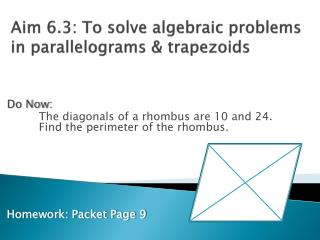Download PresentationAim 6.3: To solve algebraic problems in parallelograms & trapezoids

Aim 6.3: To solve algebraic problems in parallelograms & trapezoids - PowerPoint PPT Presentation

Aim 6.3: To solve algebraic problems in parallelograms & trapezoids. Do Now: The diagonals of a rhombus are 10 and 24.   Find the perimeter of the rhombus.  Homework: Packet Page 9. Homework Answers:. Packet page 5 SR = 12 10. Angle L = 115 RQ = 20 11. Angle T = 72I am the owner, or an agent authorized to act on behalf of the owner, of the copyrighted work described.
Download PresentationAim 6.3: To solve algebraic problems in parallelograms & trapezoids

Download Policy: Content on the Website is provided to you AS IS for your information and personal use and may not be sold / licensed / shared on other websites without getting consent from its author.While downloading, if for some reason you are not able to download a presentation, the publisher may have deleted the file from their server.

- - - - - - - - - - - - - - - - - - - - - - - - - - E N D - - - - - - - - - - - - - - - - - - - - - - - - - -
Presentation Transcript
1. Aim 6.3: To solve algebraic problems in parallelograms & trapezoids Do Now: The diagonals of a rhombus are 10 and 24.  Find the perimeter of the rhombus.  Homework: Packet Page 9

2. Homework Answers: • Packet page 5 • SR = 12 10. Angle L = 115 • RQ = 20 11. Angle T = 72 • Angle Q = 32 12. Angle 2 = 42 • Angle R = 112 13. Angle C = 54 • x = 16 14. Angle 2 = 70 • Angle S = 104 15. Angle ADC = 141 • Angle Q = 40 • Angle P = 116 • Angle Q = 65

3. Aim 6.3: To solve algebraic problems in parallelograms & trapezoids Practice with Parallelograms: • In parallelogram ABCD, AB = 5x – 3 and CD = 15 – x. Find the value of x. • Two consecutive angles of a parallelogram measure (2x + 10)o and (x – 10)o. What is the value of x? • Find the length of a side of the rhombus whose diagonals have lengths of 12 and 16. • The measures of angles A and B of parallelogram ABCD are in the ratio of  2 : 7.  Find the measure of angle A.

4. Aim 6.3: To solve algebraic problems in parallelograms & trapezoids Example #1: EF is the median of trapezoid ABCD. BC = 36 and AD = 54.  Find EF:

5. Aim 6.3: To solve algebraic problems in parallelograms & trapezoids Example #2: ABCD is an isosceles trapezoid. AB is parallel to DC, AD = BC. If Angle DAB = 84o, find Angle CBA Also, DA = 3x + 21, DC = 2x + 10, BC = 7x + 5 Solve for x: Find DC: D C A B

6. Aim 6.3: To solve algebraic problems in parallelograms & trapezoids Example #3: • WORK is an isosceles trapezoid with legs WK and OR. • Determine the length of each leg is WK = x2 and OR = x + 56 W O K R

7. Aim 6.3: To solve algebraic problems in parallelograms & trapezoids Example #1: LMNO is a parallelogram. Angle M = 110o. Find the other angles: L M N P O

8. Aim 6.3: To solve algebraic problems in parallelograms & trapezoids Example #5: PRHS is an Isosceles Trapezoid: Leg PS = 5x + 4, base SH = 9x + 8 and leg RH = 2x + 16. Determine the length of all 3 sides: P R S H

9. Aim 6.3: To solve algebraic problems in parallelograms & trapezoids Example #6: Angle G and Angle M are in a ratio of 7:5 Find Angle M M G T W

10. Aim 6.3: To solve algebraic problems in parallelograms & trapezoids Example #7: GW = 3x + 21, TW = 2x + 10, MT = 7x + 5 Find TW: M G T W

11. Aim 6.3: To solve algebraic problems in parallelograms & trapezoids Challenge: In rectangle ABCD, the diagonals intersect at E. If AE = 3x + y, BE = 4x - 2y, and CE = 20, find x and y:

12. Aim 6.3: To solve algebraic problems in parallelograms & trapezoids Exit Ticket: • True or False: Every square is a rhombus • All trapezoid has one pair of sides. • Solve for x: 12 X 35